Courses
Courses for Kids
Free study material
Free LIVE classes
More

# Half Reaction Method | JEE Main Important TopicLIVE
Join Vedantu’s FREE Mastercalss

## Redox Reactions in Everyday Life

When we cut an apple, it turns brown in colour after some time. Do you know what is the reason behind this colour change? It is because of a chemical reaction that is called oxidation. We come across oxidation reactions in our day-to-day life. For example 1) burning of LPG gas stove 2) rusting of iron 3) Oxidation of carbohydrates, lipids, etc. into Carbon dioxide and Water to produce energy in the living organisms.

Generally, all oxidation reactions are accompanied by reduction reactions and vice versa. Such reactions are called redox reactions. As per the classical concept, the addition of oxygen (or) removal of hydrogen is called oxidation, and the reverse is called reduction.

## Redox Reactions

Consider the following reactions:

Reaction 1 : $4 \mathrm{Fe}+3 \mathrm{O}_{2} \rightarrow 2 \mathrm{Fe}_{2} \mathrm{O}_{3}$

Reaction 2 : $\mathrm{H}_{2} \mathrm{~S}+\mathrm{Cl}_{2} \rightarrow 2 \mathrm{HCl}+\mathrm{S}$

Both of these two reactions are considered to be oxidation reactions as per the classical concept. In the first reaction, which shows rusting of the iron, oxygen is added to the iron. In the second reaction, the hydrogen atom is removed from Hydrogen sulphide (H2S). Determine which species will be reduced.

Consider the following two reactions given below, in which the removal of oxygen, and addition of hydrogen take place, respectively. These two reactions are called reduction reactions as per the classical concept.

$\mathrm{CuO}+\mathrm{C} \rightarrow \mathrm{Cu}+\mathrm{CO}$ (Removal of oxygen atom (O) from cupric oxide)

$\mathrm{S}+\mathrm{H}_{2} \rightarrow \mathrm{H}_{2} \mathrm{~S}$ (Addition of hydrogen atom (H2) to sulphur).

Oxidation-reduction reactions, i.e., redox reactions, are not always associated with only oxygen or only hydrogen. In such cases, the process can be explained by means of electrons. The reaction involving the loss of electrons is known as oxidation. On the other hand, the gain of electrons is coined as reduction. For example, $\mathrm{Fe}^{2+} \rightarrow \mathrm{Fe}^{3+}+e^-$ (since an electron is lost, it is oxidation). $\mathrm{Cu}^{2++} 2 e^{-} \rightarrow \mathrm{Cu}$ (since an electron is gained it is a reduction) Redox reactions can be better explained using oxidation numbers.

## Oxidation Number

Oxidation number is defined as the imaginary charge that is left on the atom when all  the other atoms of the compound have been removed in their usual oxidation states that are assigned according to a set of rules. Oxidation state is a term that is often used interchangeably with oxidation number.

## Types of Redox Reactions

Combination Reactions:

When two substances combine to form a single compound in a redox reaction are called combination reactions.

Example: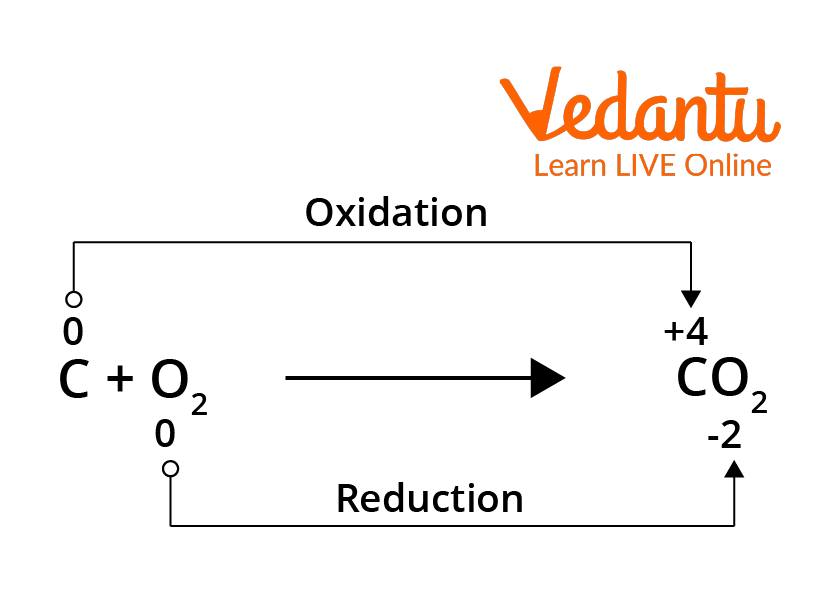Combination Reaction

Decomposition Reactions:

Redox reactions in which a compound splits up into two or more components are called decomposition reactions. These reactions are opposite to that of the combination reactions. In these reactions, the oxidation number of the different elements in the same substance will be changed.

Example: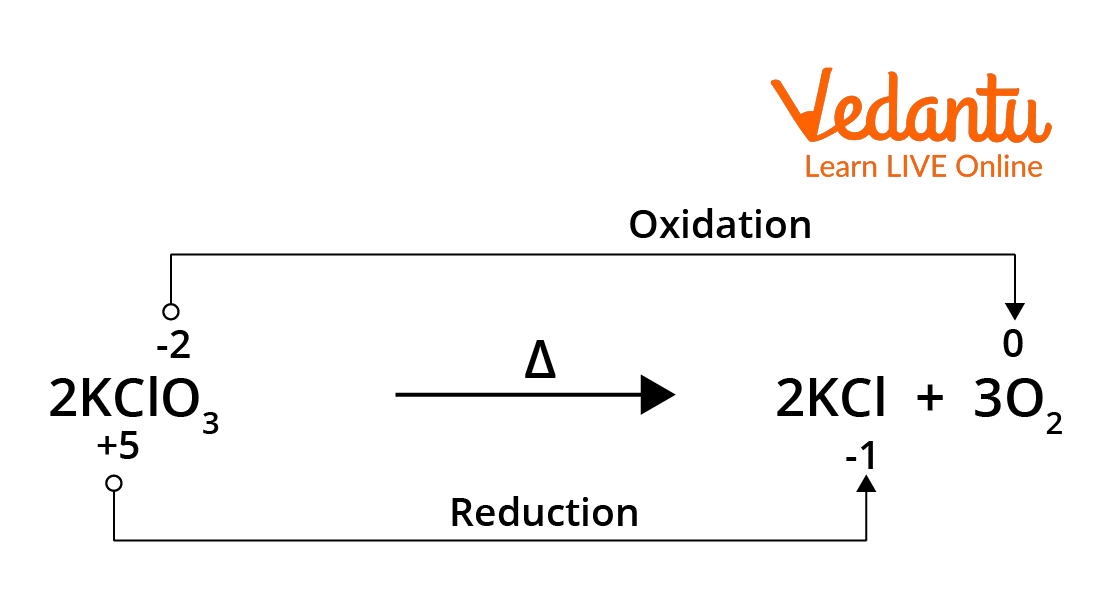Decomposition Reaction

Displacement Reactions:

In some Redox reactions, an ion (or an atom) in a compound is replaced by an ion (or atom) of another element; this type of redox reactions are called displacement reactions. These are further subdivided into two types, namely metal displacement and non-metal displacement reactions.

Example: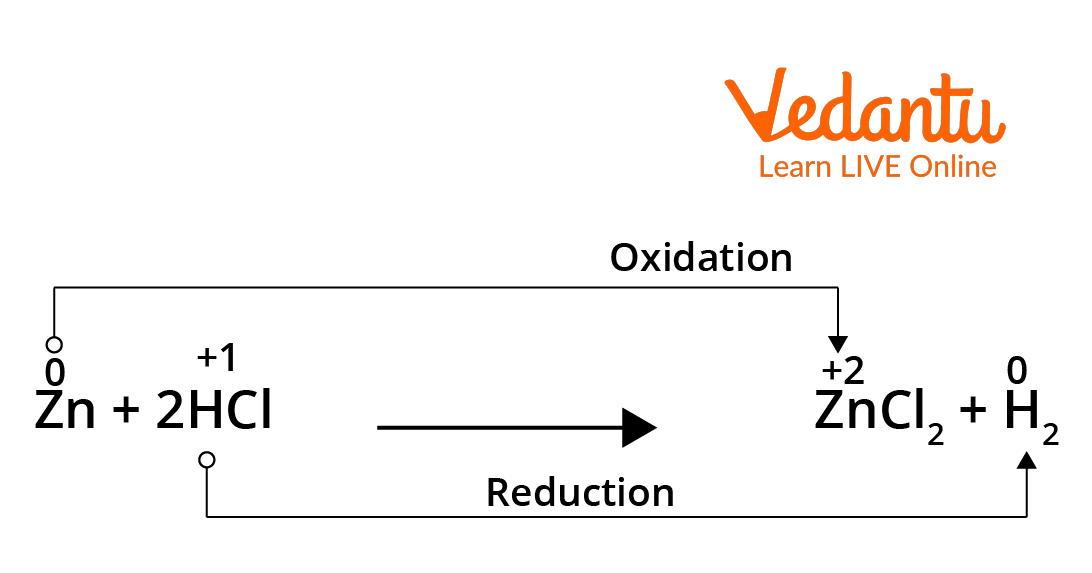Displacement Reaction

Disproportionation Reactions (Auto Redox Reactions):

In this type of redox reaction, the same compound can undergo both oxidation and reduction. Here, the oxidation state of one and the same element is both increased and decreased. These reactions are called disproportionation reactions.

Example: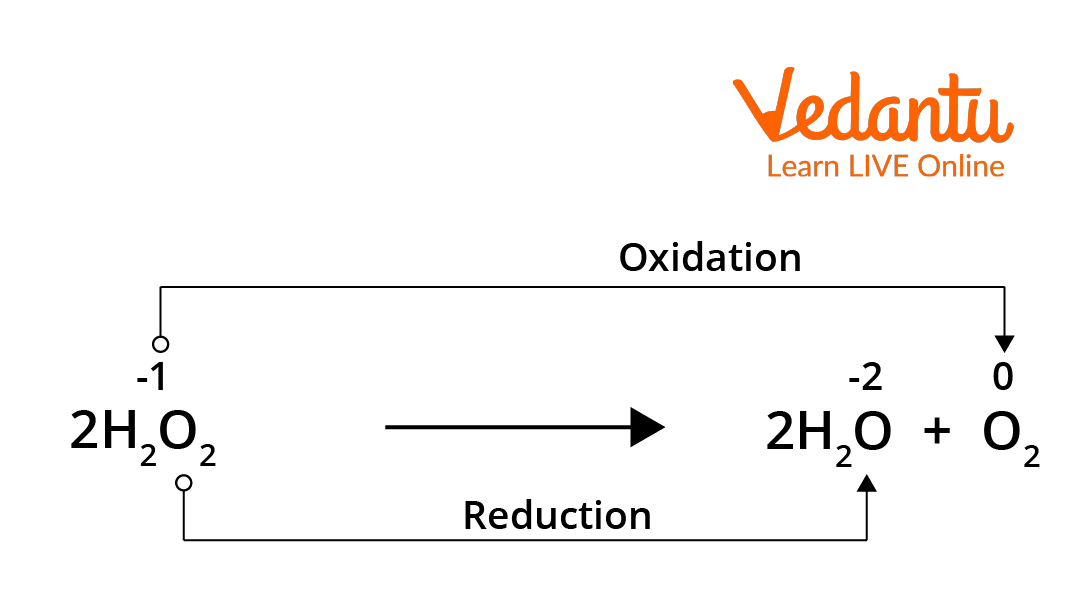Disproportionation Reaction

## Balancing Redox Reactions

To balance the equations of redox reactions, two methods are followed. They are

i) The oxidation number method

ii) Ion-electron method / half reaction method.

Both the methods are based on the fact that, in the oxidation-reduction reactions, the total number of electrons that are donated by the reducing agent will be equal to the total number of electrons that are gained by the oxidising agent.

## Balancing Redox Reaction by Half Reaction Method

This method is used for balancing the ionic redox reactions.

Step 1: Using the oxidation number concept, find out the reactants which undergo both oxidation and reduction.

Step 2: Write two separate half equations for both the oxidation half reaction and reduction half reaction. Let us consider an example of the reaction between KMNO4, FeSO4 and H2SO4.

$\mathrm{KMnO}_{4}+\mathrm{FeSO}_{4}+\mathrm{H}_{2} \mathrm{SO}_{4} \rightarrow \mathrm{MnSO}_{4}+\mathrm{Fe}_{2}\left(\mathrm{SO}_{4}\right)_{3}+\mathrm{K}_{2} \mathrm{SO}_{4}+\mathrm{H}_{2} \mathrm{O}$

The ionic form of this reaction is,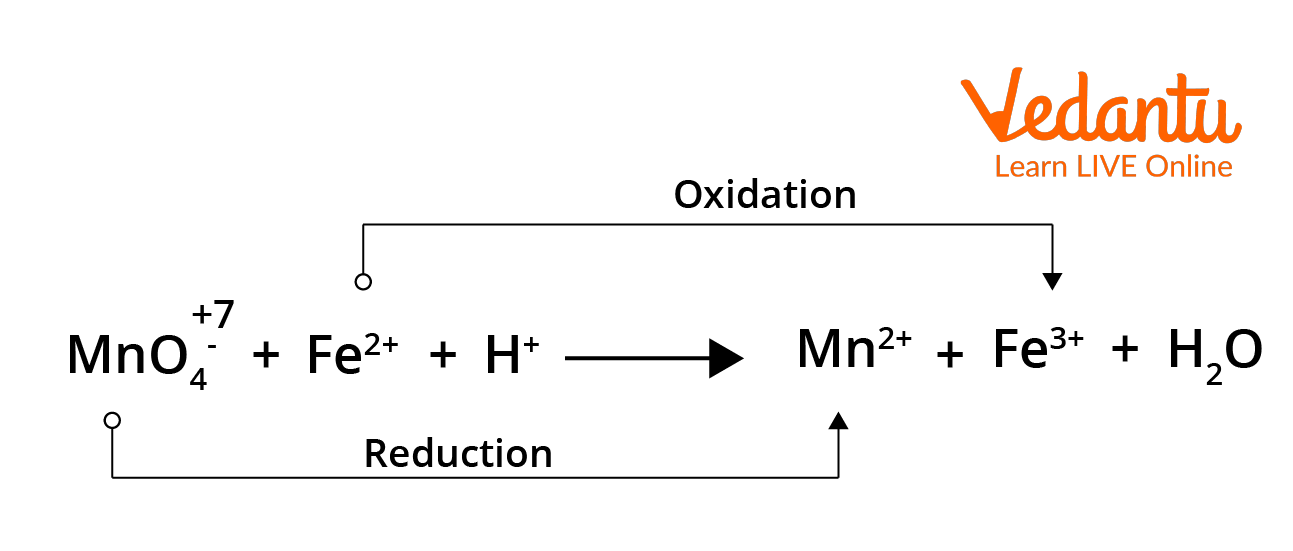Ionic form of the Redox Reaction

The two half reactions for the above reaction can be written as,

$\mathrm{Fe}^{2+} \rightarrow \mathrm{Fe}^{3+}+1{\mathrm{e}^-}$ (1) and

$\mathrm{MnO}_{4}^{-}+5 \mathrm{e}^{-} \rightarrow \mathrm{Mn}^{2+}$ (2)

Balance the atoms and charges on both sides of the above two half reactions.

Equation (1) $\Rightarrow$ No changes i.e., $\mathrm{Fe}^{2+} \rightarrow \mathrm{Fe}^{3+}+1 \mathrm{e}^{-}$  (1)

Equation (2) $\Rightarrow$ 4'O' is present on the reactant side, therefore we should add 4H2O on the product side, to balance Hydrogen atoms - add, 8H+ in the reactant side, so that the equation will be balanced.

$\mathrm{MnO}_{4}^{-}+5 \mathrm{e}^{-}+8 \mathrm{H}^{+} \rightarrow \mathrm{Mn}^{2+}+4 \mathrm{H}_{2} \mathrm{O}$  (3)

Step 3: Equate both half reactions such that the number of electrons lost is equal to the number of electrons that are gained. Addition of two half reactions gives the balanced equation that is represented by equation numbered (6).

$(1)\times 5 \Rightarrow\quad\quad\quad5 \mathrm{Fe}^{2+} \rightarrow 5 \mathrm{Fe}^{3+}+{5 \mathrm{e}^{-}}\dots \dots \dots(4)$

$(3) \times 1 \Rightarrow\quad\quad \mathrm{MnO}_{4}^{-}+{5 \mathrm{e}^-}+8 \mathrm{H}^{+} \rightarrow \mathrm{Mn}^{2+}+4 \mathrm{H}_{2} \mathrm{O}\dots \dots \dots(5)$

$(4)+(5) \Rightarrow \quad\quad5 \mathrm{Fe}^{2+}+\mathrm{MnO}_{4}^{-}+8 \mathrm{H}^{+} \rightarrow 5 \mathrm{Fe}^{3+}+\mathrm{Mn}^{2+}+4 \mathrm{H}_{2} \mathrm{O} \dots \dots \dots(6)$

So, finally $5 \mathrm{Fe}^{2+}+\mathrm{MNO}_{4}^{-}+8 \mathrm{H}^{+} \rightarrow 5 \mathrm{Fe}^{3+}+\mathrm{Mn}^{2+}+4 \mathrm{H}_{2} \mathrm{O}$ is the required balanced equation.

## Conclusion

Addition of oxygen ion (or) removal of hydrogen ion is called oxidation, and the reverse of the same is called reduction. Reactions in which both oxidation and reduction take place at the same time are called redox reactions. They are classified into combination reactions, decomposition reactions, displacement reactions, and disproportionation reactions.

To balance the redox reactions, two methods are followed, one is the half reaction and the other one is the oxidation number method. To balance the equation in the half reaction method, we have to find the reactants that have undergone both the oxidation and reduction phenomenon. Then, we have to separate both the half reactions and balance them. Then we should equate both the equations such that the number of electrons gained equals the number of electrons lost. After equating, we are required to add both the half reactions to obtain the required balanced equation.

Last updated date: 26th Sep 2023
Total views: 1.5k
Views today: 0.01k

## FAQs on Half Reaction Method | JEE Main Important Topic

1. In metal displacement reactions, Zinc replaces Copper from Copper Sulphate (CUSO4) solution. Examine whether the reverse reaction takes place or not.

As discussed earlier, place a metallic copper strip inside the zinc sulphate solution. If copper replaces the zinc atoms from the zinc sulphate solution, Cu2+ ions would be released into the solution and, as a result, the colour of the solution would change to blue. But no such change is observed. Therefore, we conclude that among zinc and copper, zinc has more tendency to release electrons while copper has a tendency to accept electrons. So from this, we can understand that the reverse reaction does not occur for the above-mentioned reaction.

2. What are Redox reactions in terms of oxidation numbers?

During the redox reactions, the oxidation numbers of the elements will change from their original values. A reaction in which the oxidation number of the element increases is known as an oxidation reaction. Meanwhile, a reaction where the oxidation number decreases is called a reduction reaction.

Example: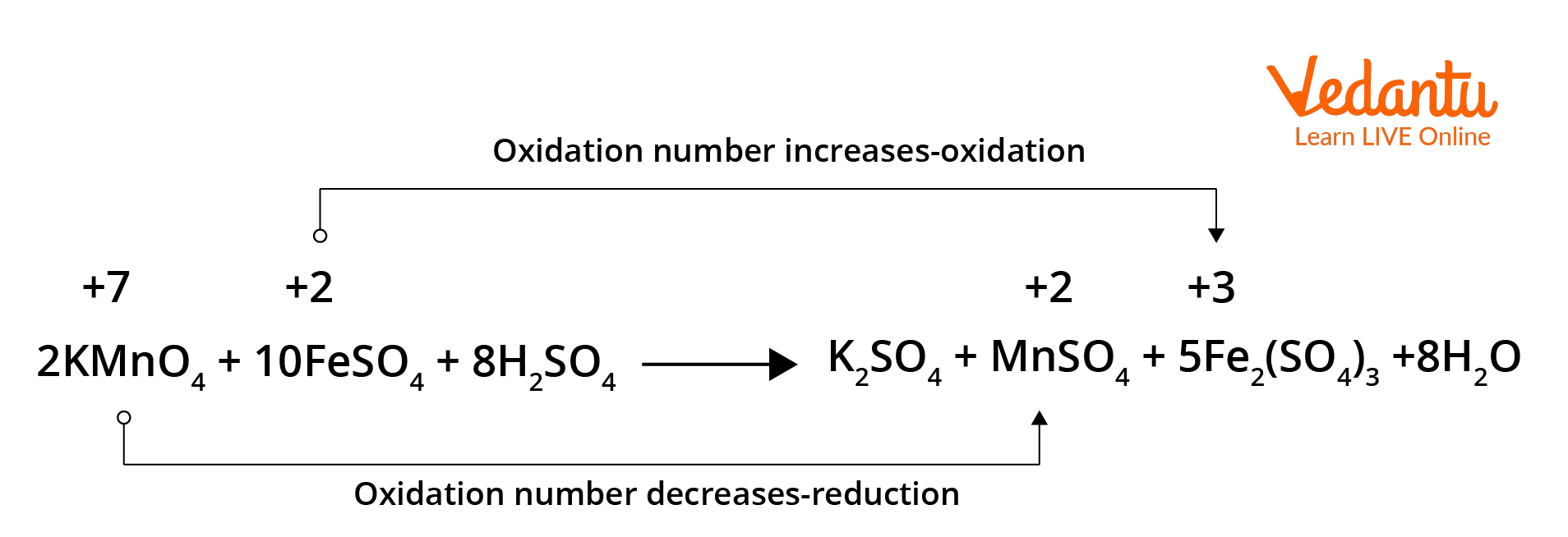Redox reaction in terms of oxidation numbers.

In the above given reaction, manganese in potassium permanganate (KMnO4) favours the oxidation of ferrous sulphate (FeSO4) into ferric sulphate (Fe2(SO4)3 by gaining electrons and thereby getting reduced. Such reagents are called oxidising agents or oxidants. Similarly, the reagents that facilitate reduction reactions by releasing electrons and getting oxidised are called reducing agents.